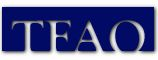The TFAO Digital Library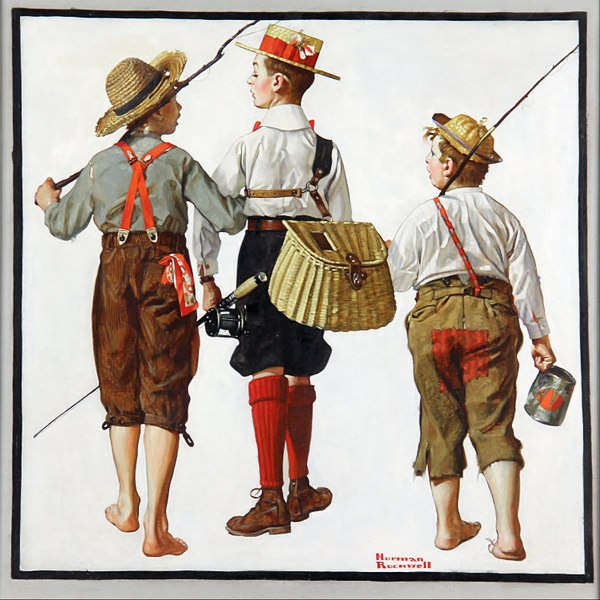(above: Norman Rockwell, Fishing Trip, They'll Be Coming Back Next Week, 1919, oil on canvas, 29 x 29 inches, Norman Rockwell Museum. Public domain, via Wikimedia Commons*)

On 11/2122 the online TFAO Digital Library had these file counts:

Text:

1aa = 701
2aa = 687
3aa = 705
4aa = 706
5aa = 683
6aa = 693
7aa = 562
8aa = 688
9aa = 671
10aa = 689
11aa = 685
12aa = 4

apprais = 160
andres = 8
anne = 12
states = 7
beserra = 32
subjects = 57
book = 3
bookpage = 172
calendar = 50
chronology = 8
distingu = 55
msjc = 8
newsassn = 76
newsm1 = 703
newsmu = 669
permc = 58
srcbook = 18
utah = 10
site = 44

Text total = 9,655

Image:

1am = 641
2am = 629
3am = 699
4am = 698
5am = 698
6am = 698
7am = 695
8am = 687
9am = 631
10am = 683
11am = 684
12am = 685
13am = 677
14am = 686
15am = 244
16am = 678
17am = 685
18am = 368
19am = 117

am series subtotal = 11,583

1cm = 799
2cm = 727
3cm = 698
4cm = 895
5cm = 667
6cm = 638
7cm = 695
8cm = 528
9cm = 697
10cm = 698
11cm = 629

cm series subtotal = 7,709

okeeffe = 14
permc = 67
srcbook = 30
utah = 11
canyon = 36
distingu = 453
enman = 15
logo = 118
lrsmith = 17
mdixon = 24
mi = 719
mi1 = 36
mn = 697
mia = 241
mib = 450
mic = 499
mid = 132
msjc = 18
musimage = 698
newsassn = 110
nmtrip = 12
apprais = 144
book = 56

miscellaneous subtotal = 4,593

Image total = 23,885

Grand total of text and image files = 33,540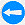Return to Content and paper-printed book equivalence.

Go to

Links to sources of information outside of our web site are provided only as referrals for your further consideration. Please use due diligence in judging the quality of information contained in these and all other web sites. Information from linked sources may be inaccurate or out of date. TFAO neither recommends or endorses these referenced organizations. Although TFAO includes links to other web sites, it takes no responsibility for the content or information contained on those other sites, nor exerts any editorial or other control over them. For more information on evaluating web pages see TFAO's General Resources section in Online Resources for Collectors and Students of Art History.

*Tag for expired US copyright of object image: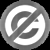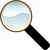Search Resource Library